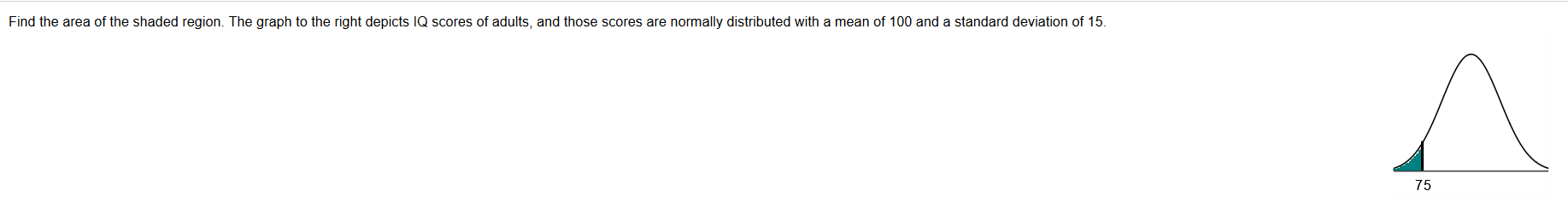Find the area of the shaded region. The graph to the right depicts IQ scores of adults, and those scores are normally distributed with a mean of 100 and a standard deviation of 15.75

Questionhelp_outlineImage TranscriptioncloseFind the area of the shaded region. The graph to the right depicts IQ scores of adults, and those scores are normally distributed with a mean of 100 and a standard deviation of 15. 75 fullscreen
Step 1

Solution

To find: The area under the shaded region of the given normal curve. i.e., area below X = 75.

Given: The IQ scores of adults are normally distributed with a mean µ = 100 and standard deviation σ = 15.

Step 1:

To find the area below X = 75, we need to find the z score corresponding to X = 75.

The z score is computed using the formula,

Step 2

Step 2:

Substitute the values X = 75, µ = 100, and σ = 15 in th...

Want to see the full answer?

See Solution

Want to see this answer and more?

Our solutions are written by experts, many with advanced degrees, and available 24/7

See Solution
Tagged in

Statistics